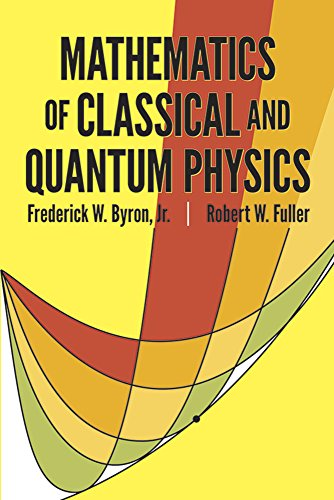# Mathematics of Classical and Quantum Physics

• Length: 661 pages
• Edition: R
• Publisher:
• Publication Date: 1992-08-20
• ISBN-10: 048667164X
• ISBN-13: 0800759671649
4.3
Description

This textbook is designed to complement graduate-level physics texts in classical mechanics, electricity, magnetism, and quantum mechanics. Organized around the central concept of a vector space, the book includes numerous physical applications in the body of the text as well as many problems of a physical nature. It is also one of the purposes of this book to introduce the physicist to the language and style of mathematics as well as the content of those particular subjects with contemporary relevance in physics.

Chapters 1 and 2 are devoted to the mathematics of classical physics. Chapters 3, 4 and 5 — the backbone of the book — cover the theory of vector spaces. Chapter 6 covers analytic function theory. In chapters 7, 8, and 9 the authors take up several important techniques of theoretical physics — the Green’s function method of solving differential and partial differential equations, and the theory of integral equations. Chapter 10 introduces the theory of groups. The authors have included a large selection of problems at the end of each chapter, some illustrating or extending mathematical points, others stressing physical application of techniques developed in the text.

Essentially self-contained, the book assumes only the standard undergraduate preparation in physics and mathematics, i.e. intermediate mechanics, electricity and magnetism, introductory quantum mechanics, advanced calculus and differential equations. The text may be easily adapted for a one-semester course at the graduate or advanced undergraduate level.